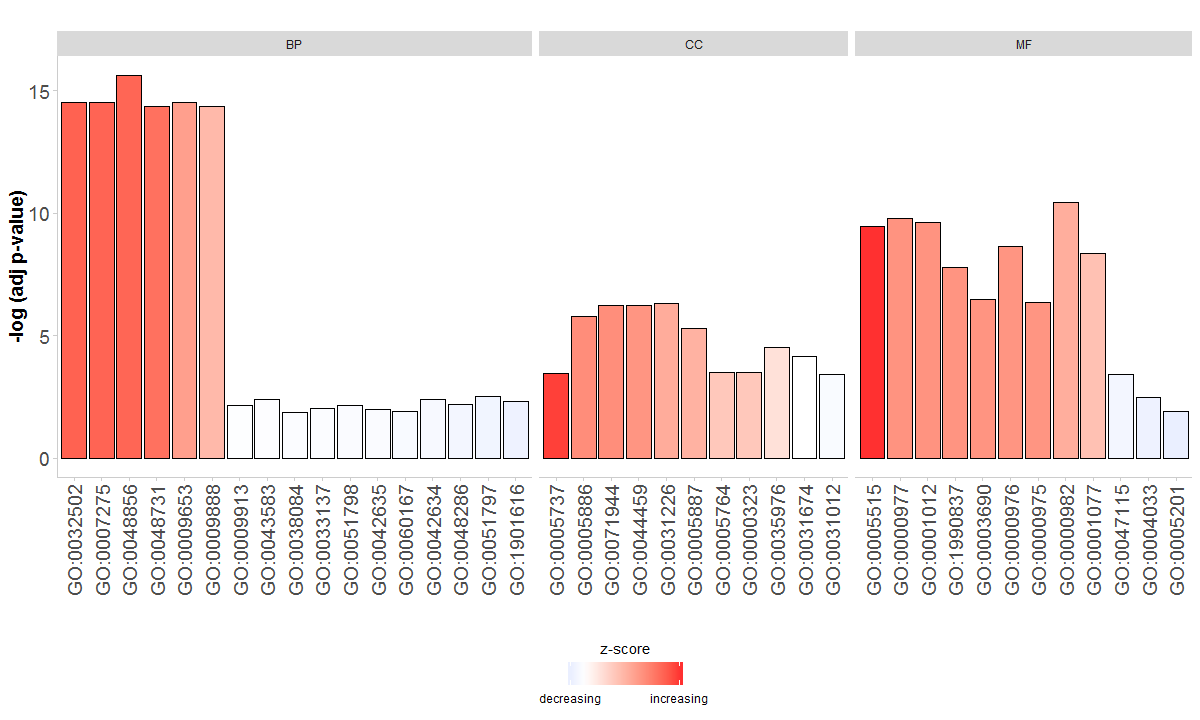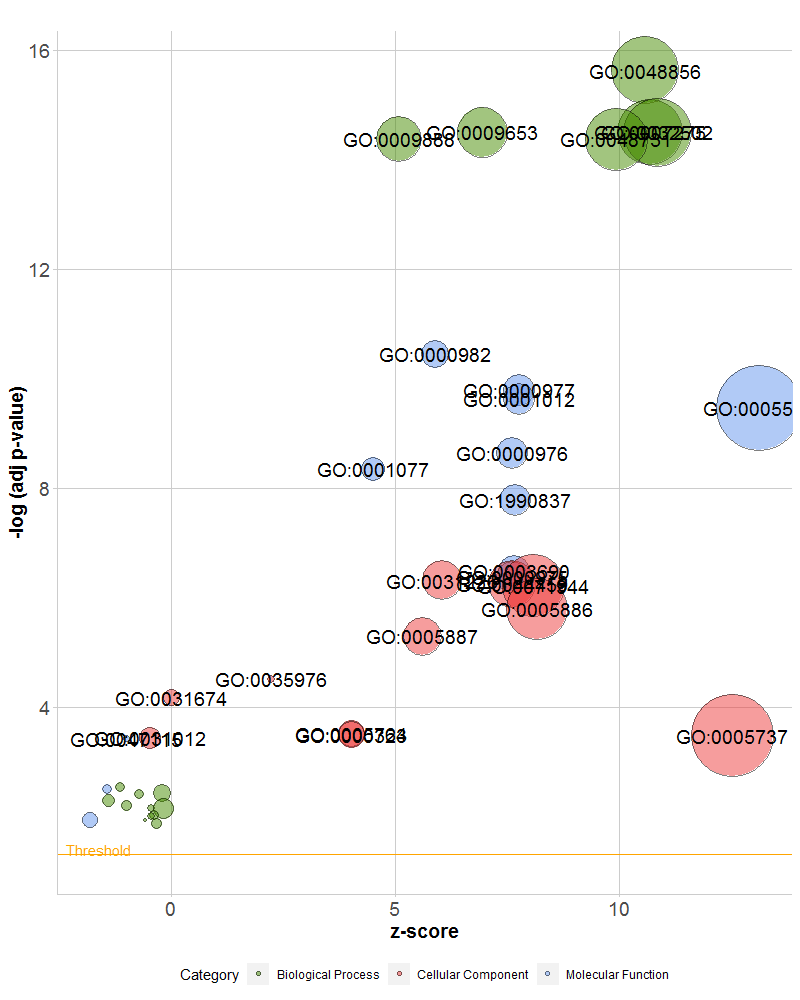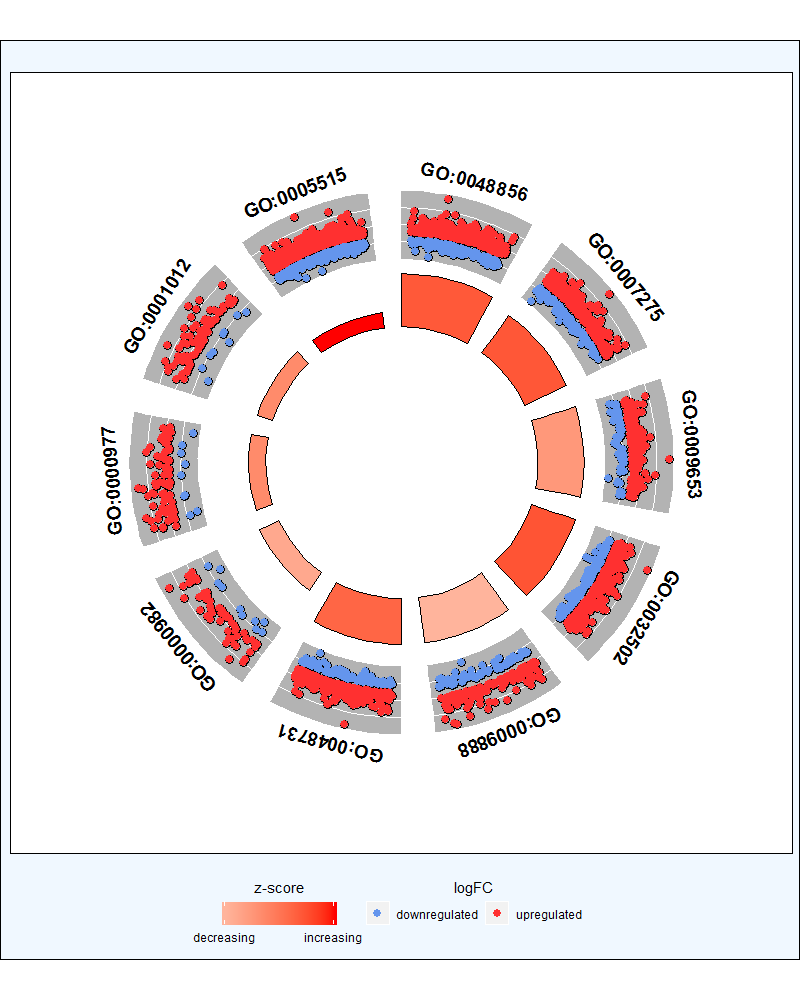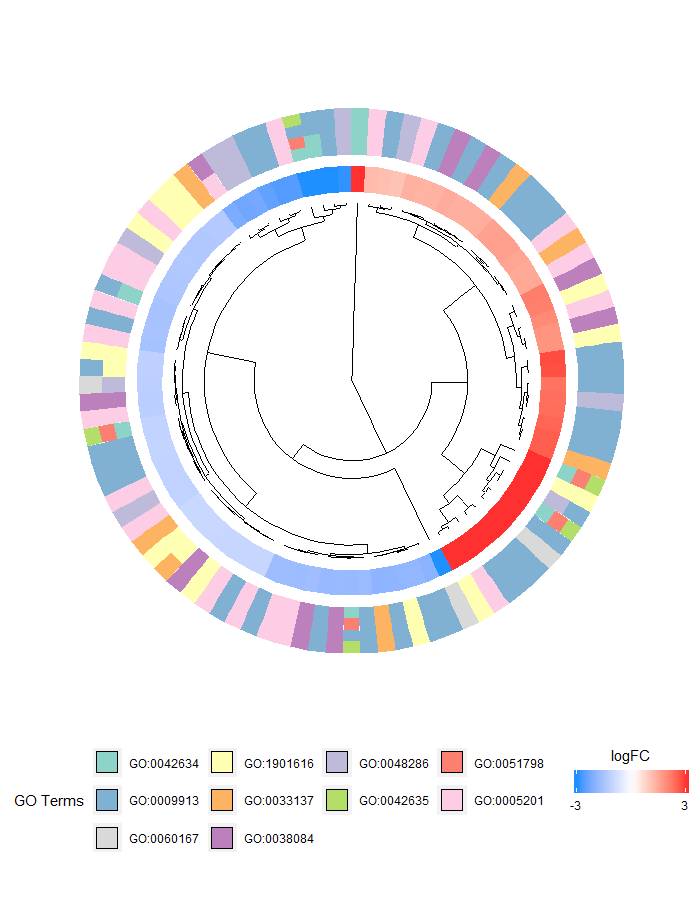## GO富集可视化-GOplot

GOplot使用了zscore概念，其并不是指Z-score标准化，而是用于表示参与某个GO Term下基因的上下调情况，公式：$zscore=\frac{(up-down)}{\sqrt{count}}$

zscore is an easy to calculate value to give you a hint if the biological process (/molecular function/cellular components) is more likely to be decreased (negative value) or increased (positive value)

GOplot使用说明可以参照官方文档：https://wencke.github.io/，其对于这个R包的使用讲的非常的详细，看一遍就懂了

library(GOplot)
deg <- read.table(file = "../Desktop/DEG.txt", sep = "\t", header = T, stringsAsFactors = F)
enrich <- read.table(file = "../Desktop/enrichment.txt", sep = "\t", header = T, stringsAsFactors = F)
circ <- circle_dat(enrich, deg)


GOBar(circ, display = 'multiple')GOBubble(circ, display = "single", labels = 3, table.legend = F)GOplot为了过滤一些比较相似的GO ID（认为是冗余的），其根据不同GO之间参与基因的相似程度进行的过滤，这个可以自己写代码预先过滤下，也可以直接用其写好的函数reduce_overlap

reduced_circ <- reduce_overlap(circ, overlap = 0.75)
GOBubble(reduced_circ, labels = 3, table.legend = F)


GOCircle(circ, nsub = 10, label.size = 5, rad1 = 3, rad2 = 4, table.legend = F)process <- tail(enrich\$ID, 10)
chord <- chord_dat(data = circ, genes = deg, process = process)
GOChord(chord, space = 0.02, gene.order = 'logFC', gene.space = 0.25, gene.size = 2.5, limit = c(0,2))GOHeat(chord, nlfc = 1, fill.col = c('red', 'yellow', 'green'))GOCluster(circ, process, clust.by = 'logFC', term.width = 2)GOplot这个R包的一些展示形式值得我们学习（因为有些绘图细节GOplot处理的不是太好，可选择参数有限），而且可以用于其他方向，不局限于GO富集；这个R包也发表了不错的文章